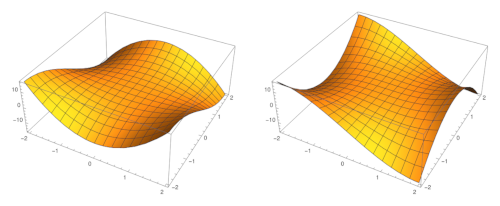# Finding the imaginary part of an analytic function from the real part

A function f of a complex variable z = xiy can be factored into real and imaginary parts:where x and y are real numbers, and u and v are real-valued functions of two real values.

Suppose you are given u(x, y) and you want to find v(x, y). The function v is called a harmonic conjugate of u.

## Finding v

If u and v are the real and imaginary parts of an analytic function, then u and v are related via the Cauchy-Riemann equations. These are first order differential equations that one could solve to find u given v or v given u. The approach I’ll present here comes from  and relies on algebra rather than differential equations.

The main result from  isSo given an expression for u (or v) we evaluate this expression at z/2 and z/2i to get an expression for f, and from there we can find an expression for v (or u).

This method is simpler in practice than in theory. In practice we’re just plugging (complex) numbers into equations. In theory we’d need to be a little more careful in describing what we’re doing, because u and v are not functions of a complex variable. Strictly speaking the right hand side above applies to the extensions of u and v to the complex plane.## Example 1

Shaw gives three exercises for the reader in . The first isWe find thatWe know that the constant term is purely imaginary because u(0, 0) = 0.

Thenand sois a harmonic conjugate for u for any real number β.

The image above is a plot of the function u on the left and its harmonic conjugate v on the right.

## Example 2

Shaw’s second example isWe begin withand soFrom there we find## Example 3

Shaw’s last exercise isThen